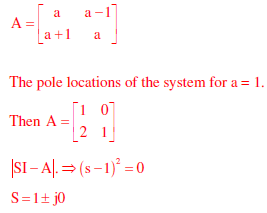# Signals and Systems - Online Test

Q1. A band-limited signal with a maximum frequency of 5 kHz is to be sampled. According to the sampling theorem, the sampling frequency in kHz which is not valid is
Explaination / Solution:
No Explaination.

Q2. For a periodic signal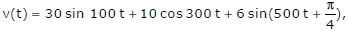the fundamental frequency in radians/s is
Explaination / Solution:
No Explaination.

Q3. A continuous random variable X has a probability density functionis
Explaination / Solution:
No Explaination.

Q4. Two systems with impulse responses h1(t) and h2(t) are connected in cascade. Then the overall impulse response of the cascaded system is given by
Explaination / Solution:
No Explaination.

Q5. The impulse response of a system is h(t) = tu(t). For an input u(t − 1), the output is
Explaination / Solution:
No Explaination.

Q6. The impulse response of a continuous time system is given by h(t) = δ(t - 1) + δ(t - 3). The value of the step response at t = 2 is
Explaination / Solution:
No Explaination.

Q7. The state variable formulation of a system is given asThe response y(t) to the unit step input is
Explaination / Solution:
No Explaination.

Q8. The state variable formulation of a system is given asThe system is
Explaination / Solution:
No Explaination.

Q9. Consider an LTI system with impulse response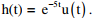If the output of the system is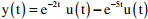then the input, x(t), is given by
Explaination / Solution: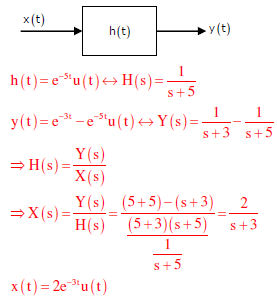Q10. A discrete system is represented by the difference equationIt has initial condition X1(0) = 1; X2(0) = 0. The pole location of the system for a = 1, are
Explaination / Solution:

from the given difference equation,